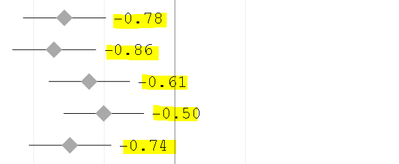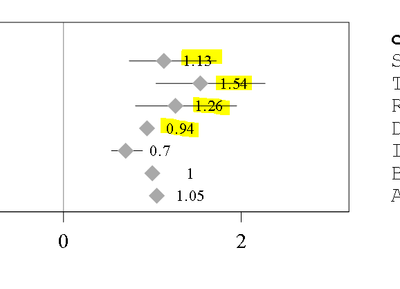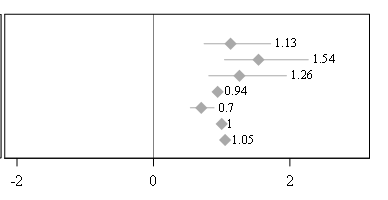## How to avoid the datalabels overlapping on Error bars in Forest Plot

I am creating the data labels on the forest plot. However My data labels overlapping with the Error bars. How I can Avoid this. I tried using DATALABELPOSITION But no luck. I thought of Creating Blank space before dataset ( data point values). However it won't change it dynamically , if any values changes in future. Can you please suggest the Options.

Example: How the labels should look!How My labels are displaying``````layout overlay /xaxisopts=(label=' ' linearopts =(tickvaluelist=(-2 0 2) viewmin=-2 viewmax=2))
/*type=discrete discreteopts =(  tickvaluelist=( '-0.5' '0' '0.5' '1' '2')))*/
y2axisopts = (display=none);
scatterplot x=OR y=ORCI /
markerattrs = (symbol=diamondfilled size=0 color=white) yaxis=y2;
scatterplot x=OR y=ORCI  / datalabel = OR datalabelattrs = (size=7) datalabelposition=right
markerattrs = (symbol=diamondfilled size=10 color=darkgrey)
yaxis=y2
xerrorlower = lcl xerrorupper = ucl errorbarcapshape = none;
referenceline x=0;
annotate;

endlayout;``````

1 ACCEPTED SOLUTION

Accepted SolutionsDanH_sas
SAS Super FREQ

## Re: How to avoid the datalabels overlapping on Error bars in Forest Plot

Instead using the error bar on the SCATTERPLOT statement, use a HIGHLOWPLOT plot. Set the LOWCAP=NONE and HIGHCAP=none, and set the HIGHLABEL to be the same variable as the DATALABEL. The change should look something like the following:

``````layout overlay /xaxisopts=(label=' ' linearopts =(tickvaluelist=(-2 0 2) viewmin=-2 viewmax=2))
/*type=discrete discreteopts =(  tickvaluelist=( '-0.5' '0' '0.5' '1' '2')))*/
y2axisopts = (display=none);
scatterplot x=OR y=ORCI / markerattrs = (symbol=diamondfilled size=0 color=white) yaxis=y2;
highlowplot y=ORCI low=lcl high=ucl / lowcap=none highcap=none highlabel=OR labelattrs=(size=7)                         lineattrs=(color=darkgrey) yaxis=y2;
scatterplot x=OR y=ORCI  /
markerattrs = (symbol=diamondfilled size=10 color=darkgrey)
yaxis=y2;
referenceline x=0;
annotate;

endlayout;``````

Hope this helps!

Dan

2 REPLIES 2DanH_sas
SAS Super FREQ

## Re: How to avoid the datalabels overlapping on Error bars in Forest Plot

Instead using the error bar on the SCATTERPLOT statement, use a HIGHLOWPLOT plot. Set the LOWCAP=NONE and HIGHCAP=none, and set the HIGHLABEL to be the same variable as the DATALABEL. The change should look something like the following:

``````layout overlay /xaxisopts=(label=' ' linearopts =(tickvaluelist=(-2 0 2) viewmin=-2 viewmax=2))
/*type=discrete discreteopts =(  tickvaluelist=( '-0.5' '0' '0.5' '1' '2')))*/
y2axisopts = (display=none);
scatterplot x=OR y=ORCI / markerattrs = (symbol=diamondfilled size=0 color=white) yaxis=y2;
highlowplot y=ORCI low=lcl high=ucl / lowcap=none highcap=none highlabel=OR labelattrs=(size=7)                         lineattrs=(color=darkgrey) yaxis=y2;
scatterplot x=OR y=ORCI  /
markerattrs = (symbol=diamondfilled size=10 color=darkgrey)
yaxis=y2;
referenceline x=0;
annotate;

endlayout;``````

Hope this helps!

Dan

## Re: How to avoid the datalabels overlapping on Error bars in Forest Plot

Perfect. It worked. Thanks Dan.😍Discussion stats
• 2 replies
• 641 views
• 4 likes
• 2 in conversation